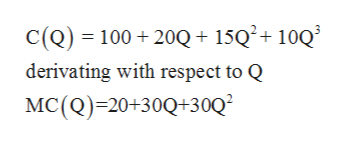An economist estimated that the cost function of a single-product firm is:                                                             C(Q) = 100 + 20Q + 15Q2 + 10Q3                             [NOTE à MC(Q) = 20+30Q+30Q2]                Based on this information, determine:The marginal cost when Q = 10

Question

An economist estimated that the cost function of a single-product firm is:

C(Q) = 100 + 20Q + 15Q2 + 10Q3

[NOTE à MC(Q) = 20+30Q+30Q2]

Based on this information, determine:

1. The marginal cost when Q = 10
Step 1

Marginal Cost: It refers to the additional cost incurred when one more unit of a good is produced.

Step 2

The total cost function is given as follows:

Step 3

The marginal cost function can be found by deriv...help_outlineImage TranscriptioncloseC(Q) 100 20Q+ 15Q2+ 10Q derivating with respect to Q MC(Q) 20+30Q+30Q2 fullscreen

Want to see the full answer?

See Solution

Want to see this answer and more?

Our solutions are written by experts, many with advanced degrees, and available 24/7

See Solution
Tagged in